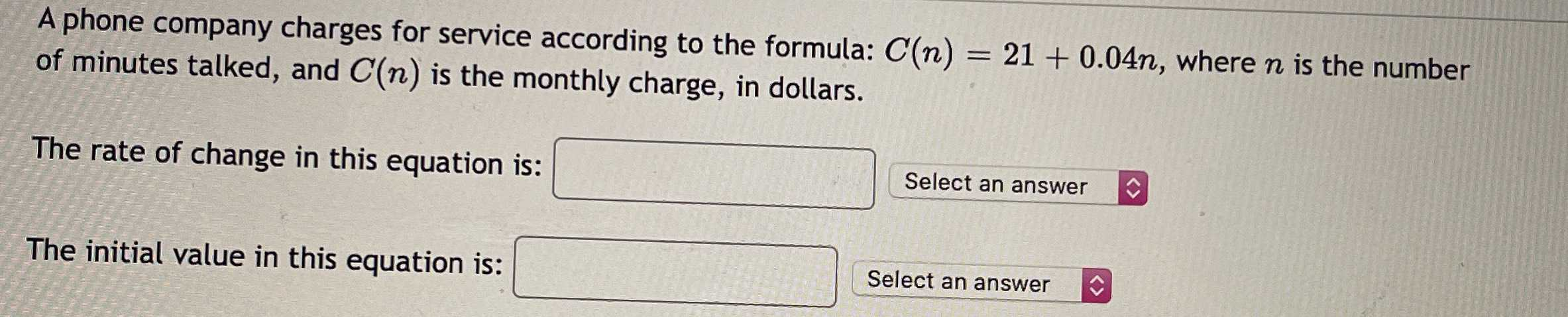### ¿Todavía tienes preguntas de matemáticas?

Pregunte a nuestros tutores expertos
Algebra
PreguntaA phone company charges for service according to the formula: $$C ( n ) = 21 + 0.04 n ,$$ where $$n$$ is the number of minutes talked, and $$C ( n )$$ is the monthly charge, in dollars.

The rate of change in this equation is: _______?

The initial value in this equation is: ______?

$$0.04\\21$$